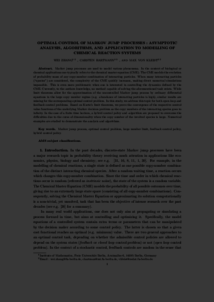Repository: Freie Universität Berlin, Math Department

# Optimal control of Markov jump processes: Asymptotic analysis, algorithms, and application to modelling of chemical reaction systems

Zhang, W. and Hartmann, C. and von Kleist, M. (2018) Optimal control of Markov jump processes: Asymptotic analysis, algorithms, and application to modelling of chemical reaction systems. Communications in Mathematical Sciences, 16 (2). pp. 293-331. ISSN 1539-6746Preview
PDF - Submitted Version
728kB

## Abstract

Markov jump processes are used to model various phenomena. In the context of biological or chemical applications one typically refers to the chemical master equation (CME). The CME models the evolution of probability mass of any copy-number combination of interacting particles. When many interacting particles (“species”) are considered, the complexity of the CME quickly increases, making direct numerical simulations impossible. This is even more problematic when one is interested in controlling the dynamics defined by the CME. Currently, to the authors knowledge, no method capable of solving the aforementioned task exists. While limit theorems allow for the approximation of the uncontrolled Markov jump process by ordinary differential equations in the large copy number regime (e.g. abundance of interacting particles is high), similar results are missing for the corresponding optimal control problem. In this study, we address this topic for both open loop and feedback control problems. Based on Kurtz’s limit theorems, we prove the convergence of the respective control value functions of the underlying Markov decision problem as the copy numbers of the interacting species goes to infinity. In the case of a finite time horizon, a hybrid control policy and -algorithm are proposed to overcome the difficulties due to the curse of dimensionality when the copy number of the involved species is large. Numerical examples are studied to demonstrate the analysis and algorithms.

Item Type: Article Mathematical and Computer Sciences > Mathematics > Mathematical Modelling Department of Mathematics and Computer Science > Institute of Mathematics > BioComputing Group 1760 Carsten Hartmann 02 Dec 2015 16:20 12 Mar 2019 12:15

Repository Staff Only: item control page